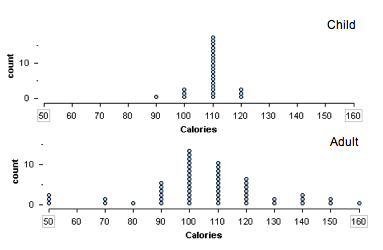## Standard Deviation (2 of 4)

### Learning Objectives

• Use mean and standard deviation to describe a distribution.

### A More Common Measure of Spread about the Mean: The Standard Deviation

The standard deviation (SD) is a measurement of spread about the mean that is similar to the average deviation. We think of standard deviation as roughly the average distance of data from the mean. In other words, the standard deviation is approximately equal to the average deviation. We develop the formula for standard deviation in the following example.

## Calculating the Standard Deviation

Let’s consider the same data set we used on the previous page: 2, 2, 4, 5, 6, 7, 9. We already know that the mean is 5. We compute the standard deviation similarly to the way we compute the average deviation. We begin by computing the deviation of each point from the mean, but instead of taking the absolute value of the differences, we square them. Here are the steps:

1. We start by finding the differences between each value and the mean (just like before):$\begin{array}{l}2-5=-3\\ 2-5=-3\\ 4-5=-1\\ 5-5=0\\ 6-5=1\text{}\\ 7-5=2\text{}\\ 9-5=4\end{array}$
2. We square each of the differences:$\begin{array}{l}{(2-5)}^{2}={(-3)}^{2}=9\\ {(2-5)}^{2}={(-3)}^{2}=9\\ {(4-5)}^{2}={(-1)}^{2}=1\\ {(5-5)}^{2}={0}^{2}=0\\ {(6-5)}^{2}={1}^{2}=1\\ {(7-5)}^{2}={2}^{2}=4\\ {(9-5)}^{2}={4}^{2}=16\end{array}$
3. As before, we find the average of these squared differences. We add the squared differences and divide by n − 1 (the count minus 1). Note that we divide by n − 1 instead of n. (The reason is subtle. We do not discuss it in this course.)$\frac{9+9+1+0+1+4+16}{6}=\frac{40}{6}\approx 6.67$
4. To scale back the value to account for the squaring we did in step 2, we take the square root of the value we found in step 3:$\sqrt{6.67}\approx 2.58$

Notice that the standard deviation is a little bit larger than the average deviation (which was 2). We can get a good approximation of the standard deviation by estimating the average distance from the mean. The shaded box on the following dotplot indicates 1 SD to the right and left of the mean.### Comment

The formula for the standard deviation of a data set can be described by the following expression. However, we will always use technology to perform the actual computation of the standard deviation.

$\sqrt{\frac{∑{(x-\stackrel{¯}{x})}^{2}}{n-1}}$

The symbols in the expression are defined as follows:

• n is the number of values in the data set (the count).
• Recall that ∑ means to add up (compute the sum).
• $\stackrel{¯}{x}$ is the mean of the data set.
• The individual values are denoted by x.

Note: In the formula you can see

• the deviations from the mean $(x-\stackrel{¯}{x})$.
• the squaring of these deviations.
• the averaging of the squared deviations: add them up (∑) and divide by (n − 1).

Before we learn to use technology to compute the standard deviation, we practice estimating it. We can estimate standard deviation in the same ways we estimated ADM. Think of standard deviation as roughly equal to ADM, so standard deviation is roughly the average distance of data from the mean.

### Learn By Doing

Let’s consider the same collection of cereals we worked with previously, except this time we’ll look at the calorie content.https://assessments.lumenlearning.com/assessments/3455